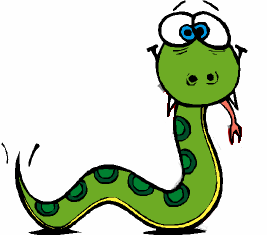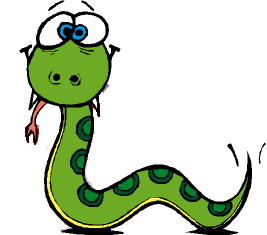Lab 6 - Python (Reloaded)Objectives
1. Review the following programming elements: if statements and string operations.
2. Practice writing Python programs

If Statements

```if <condition>:```

`<statements>`

```elif <condition>:```

`<statements>`

`else:`

`<statements>`

Example: What is the output of the following program?

```x = 9
y = 2
if x/y>4:
print "if"
else:
print "else"
print "freedom"
```

Practice I: Maximum of 3 integers

Our first task is to write a program, which asks the user to input 3 integers and outputs the maximum of them.

String Operations

Consider the following string:

`>>> x = "I am you are"`

What would the following expressions produce?

`>>> x`

`>>> x[-1]`

`>>> x[2:4] + x[6:8]`

`>>> x[:4]`

`>>> x[5:]`

`>>> x[1:2] * 3`

Given x, provide a way to access "you" with negative indices

Practice II : Strings

Creating String expressions

Execute the following statements in Python:
```    import string
str1 = "Go"
str2 = "Terriers!"
```
Now, for each of the following values, construct a Python expression involving string operations on `str1` and/or `str2` that evaluates to that value.
1.     `"Go Terriers!"`      use "+"
2.     `"GOT!"`                      use "upper"
3.     `'Torriors!'`            use "replace"

Using the "in" keyword

Consider the following expression:

```>>> "base" in "datacase"```

What is the type of this expression?

Reading strings from the terminal

Assume that the following raw_input statements are both executed:

```>>> mine=raw_input("My favorite number: ")```

```>>> yours=raw_input("Your favorite number: ")```

Write one or more statements that prints the sum of our (mine and yours) favorite numbers! (Hint: Don't forget that raw_input always gives you a string. How can you change the string to an integer?)

Printing vertically

Write a program to read a word into the variable word and print each letter of the word twice on its own line.

Example: if the user inputs "CS", the output should be:

```C C
S S
```

Splitting strings

Useful functions from the string library:

` >>> import string`

``` >>> temp= "There's four new colors in the rainbow, an old man's taking polaroids"```

` >>> parts1=string.split(temp)`

` >>> parts2=string.split(temp,",")`

` >>> temp1=string.join(parts1)`

` >>> temp2=string.join(parts2)`

Is `temp1==temp`?

What about `temp2==temp`?

What does the following command do?
`>>> string.replace(temp, " ", ";")`